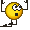# Conceptual difficulty with faradays law

DivergentSpectrum
As i understand, the induced current in a wire equals -d(∫∫B⋅dS)/dt
What is S? I know it has to be an open surface for the flux integral to be non-zero, but over what surface do i do the integral?

Mentor
As i understand, the induced current in a wire equals -d(∫∫B⋅dS)/dt
That should be the induced EMF, not current.

What is S? I know it has to be an open surface for the flux integral to be non-zero, but over what surface do i do the integral?
Consider the surface bounded by the wire.

•DivergentSpectrum
DivergentSpectrum
Oh that's right the voltage not current (i don't know why they say emf is a force if its really a potential)
so the loop has to be closed then?

Mentor
The loop has to be closed. In principle, you can use any open surface whose boundary is the loop in question. In practice, you use a surface that makes it easy to calculate the flux of ##\vec B##.

•DivergentSpectrum
DivergentSpectrum
Thanks that makes since I was just thinkin in terms of some general wire but I guess the circuit would have to be closed for you to attach a voltometer.

So if the circuit is open there is no voltage generated?

Mentor
A changing magnetic field is associated with an electric field, according to Maxwell's equations: $$\vec \nabla \times \vec E = - \frac{\partial \vec B}{\partial t}$$ or $$\oint {\vec E \cdot d \vec l} = - \frac{d}{dt} \int {\vec B \cdot d \vec a}$$ The electric field in turn produces an emf along a specified path: $$\mathcal{E} = \int {\vec E \cdot d \vec l}$$ So I would say the emf exists because the electric field does, regardless of whether there is a wire along that path or not, or a voltmeter to measure it. In order to produce a steady-state current, you need a circuit, i.e. a closed loop of wire that electrons can travel along.

•DivergentSpectrum
DivergentSpectrum
A changing magnetic field is associated with an electric field, according to Maxwell's equations: $$\vec \nabla \times \vec E = - \frac{\partial \vec B}{\partial t}$$ or $$\oint {\vec E \cdot d \vec l} = - \frac{d}{dt} \int {\vec B \cdot d \vec a}$$ The electric field in turn produces an emf along a specified path: $$\mathcal{E} = \int {\vec E \cdot d \vec l}$$ So I would say the emf exists because the electric field does, regardless of whether there is a wire along that path or not, or a voltmeter to measure it. In order to produce a steady-state current, you need a circuit, i.e. a closed loop of wire that electrons can travel along.
interesting... i wish the book i read had put it that way. i never even knew the curl of the electric field could be non-zero this really gives me a whole new perspective of what's going on here

Mentor
i never even knew the curl of the electric field could be non-zero

You started with electrostatics in which ##\vec E## does not change with time, and magnetostatics in which ##\vec B## does not change with time. In those situations, ##\vec \nabla \times \vec E = 0## so ##\vec E## is a conservative field and you can define the electric potential. Now you're starting on electrodynamics (time-varying ##\vec E## and ##\vec B## fields) in which this is no longer the case.
Pretty soon you'll see Maxwell's Equations all together in their glory.(This stuff was what made me decide to be a physics major, by the way!)

DivergentSpectrum
lol awesome i know relativity is up next so I am getting pretty excited